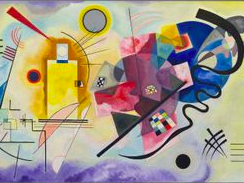## What are Relational Operators?Unlike logical operators which connect two or more expressions, relational operators test and establish some type of relation between two or more operands.

There are six (6) relational operators, which are:

1. Equal to (==)

Yes, I know what you are thinking. We should have used the well-know equal sign (=) right? Well, that falls under arithmetic operators, and in programming it is called the simple assignment operator. The equals operator checks if the values of two operands are equal or not. If yes, the condition becomes true otherwise it becomes false.

``````//Example
let a, b;
a = 3; b = 4;
console.log(a==b) //FALSE``````

2. Not Equals (!=)

This also checks if the values of two operands are equal or not. If they are not equal, the condition becomes true otherwise it becomes false.

``````//Example
let a, b;
a = 3; b = 4;
console.log(a!=b) //TRUE``````

3. Greater Than (>):

Checks if the value of the left operand is greater than the value of the right operand. If so, the condition becomes true otherwise it returns false.

``````//Example
let a, b;
a = 3; b = 4;
console.log(a>b) //FALSE``````

4. Less Than (<):

Checks if the value of the left operand is less than that of the right operand. If so, the condition becomes true, otherwise it returns false.

``````//Example
let a, b;
a = 3; b = 4;
console.log(a<b) //TRUE``````

5. Greater Than or Equals (>=)

Checks if the value of the left operand is less than or equal to the right operand. If one of them is true, the condition becomes true, otherwise it returns false.

``````//Example
let a, b;
a = 3; b = 4;
console.log(a>=b) //FALSE because a is neither greater than or equal to b``````

6. Less Than or Equals (<=)

Checks if the value of the left operand is less than or equal to the right operand. If yes, the condition becomes true. Otherwise it returns false.

``````//Example
let a, b;
a = 3; b = 4;
console.log(a<=b) //TRUE because while a is not equal to be, it is certainly less than b``````

Why you should be interested in software development

THE END.